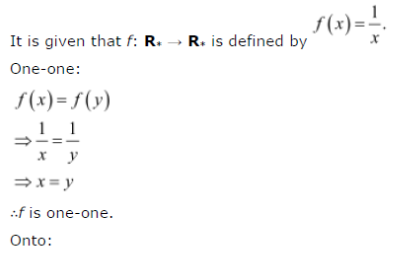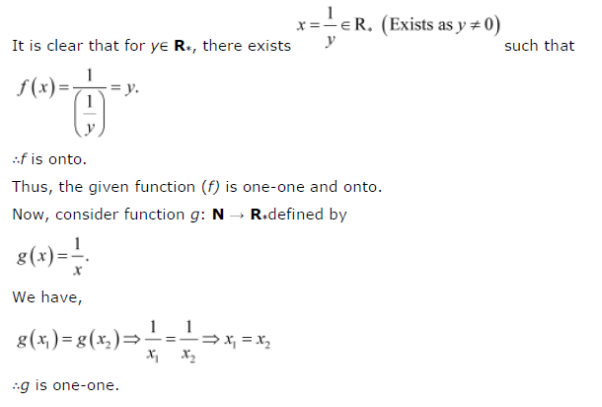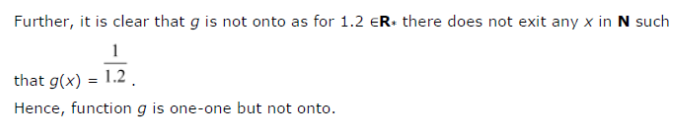# Show that the function f: R* → R* defined by f(x) = 1/x is one - one and onto, where R*

Show that the function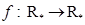defined by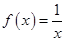is one-one and onto, where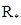is the set of all non-zero real numbers. Is the result true, if the domainis replaced by N with co-domain being same as?